# Algebraic Knot Theory - A Call for Action

## Contents

### Abstract

We knot theorists have lots of algebraically-defined knot invariants, but they tell us just little about knots. In this manifesto we suggest a reason for the failure and the means to rectify it.

In summary, we argue that we need to study knot theory within the larger context of knotted trivalent graphs and a certain collection of operations between those. With these operations, many interesting properties of knots become algebraically definable and hence susceptible to algebraic study. A required tool is a structure-respecting map from the topological world of knotted graphs and operations on those to an algebraic context. Such maps exist, with a prime example being an appropriate extension of the Kontsevich integral. But while the Kontsevich integral was thoroughly studied from other perspectives, it remains severely under-studied as a foundation for an algebraic knot theory.

### Algebraic Knot Invariants: What They Do and What They Don't

There is now a highly developed theory of knot invariants defined (or that can be defined) by algebraic means. The list contains the Alexander-Conway polynomial, the Jones polynomial and its various generalizations depending on a choice of a Lie algebra and a representation thereof, finite type invariants and the Kontsevich integral, various knot homologies and more.

These invariants are quite good at telling knots apart. While it is not known if these invariants separate knots, in practice, for the first few million knots as enumerated by computers, these invariants are either separating or they come very close to that (which one it is also depends on the precise class of invariants under consideration).

But beyond knot separation, knot theorists are interested in many other questions. Let me list just a few, with some bias in favour of the questions our still-imaginary "Algebraic Knot Theory" seems more likely to address:

1. How many crossing changes are required to unknot a given knot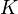$K$?
2. What is the minimal genus of a Seifert surface whose boundary is$K$?
3. Is a given link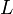$L$ a boundary link? (That is, is there a collection of disjoint Seifert surfaces for the components of$L$?)
4. Is$K$ a ribbon knot? (Recall that a ribbon knot is a knot that bounds a disk that is allowed to have "ribbon-type" singularities but is not allowed to have "clasp-type" singularities; see the image on the right).
5. Is$K$ a slice knot? That is, does$K$ bound a singularity-free disk in the four-ball?
6. Is$K$ fibered? (See an animation by Robert Barrington Leigh).
7. Does$K$ have some symmetries?
8. Is$K$ the closure of a braid on at most 6 strands?
9. Does$K$ have a projection with less than 23 crossings?
10. Does$K$ have an alternating projection?
11. Is$K$ algebraic?

With the exception of the Alexander polynomial (and its corresponding knot homology theory), currently algebraic knot invariants say very little on these questions. And while the Alexander polynomial is useful in answering some of these questions for some knots, it is simply not as strong as we wish it could be, and is not powerful enough to answer many of these questions for many other knots.

Thus as a whole, algebraically-defined knot invariants tell as very little about the knot properties we care about.

### Definability and Knotted Trivalent Graphs

Why is this so?

Take for example the property of "being a ribbon knot". This property has a lovely definition in terms of of knotted trivalent graphs and some operations between such graphs. Let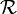${\mathcal R}$ denote the collection of all ribbon knots, and let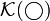${\mathcal K}(\bigcirc)$,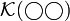${\mathcal K}(\bigcirc\bigcirc)$ and Failed to parse (unknown function\dumbbell): {\mathcal K}(\dumbbell)

denote the spaces of knotted circles, knotted pairs of circles, and knotted embeddings of the dumbbell graph Failed to parse (unknown function\dumbbell): (\dumbbell)
into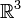${\mathbb R}^3$. Let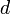$d$ and$u$ be the operations Failed to parse (unknown function\dumbbell): d:{\mathcal K}(\dumbbell)\to{\mathcal K}(\bigcirc\bigcirc)
and Failed to parse (unknown function\dumbbell): u:{\mathcal K}(\dumbbell)\to{\mathcal K}(\bigcirc)
defined by deleting and "unzipping" the middle edge of the dumbbell graph, as illustrated in the following figure:


Finally, standing by themselves, let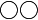$\bigcirc\bigcirc$ denote the two unknotted and unlinked circles.

Almost Theorem. The collection of all ribbon knots is equal to the collection of all unzips of knotted dumbbell whose middle deletion is the two components unlink. In symbols, Failed to parse (unknown function\dumbbell): {\mathcal R}=\{u\gamma:\gamma\in{\mathcal K}(\dumbbell)\mbox{ and }d\gamma=\bigcirc\bigcirc\} .

Semi Proof. The inclusion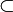$\subset$ is very easy. The other inclusion is a bit harder and also a bit false. But it is not hard to fix, and for the purpose of this quick discussion, it is better to pretend that it is true.

Let us get back now to the failure of most algebraic knot invariants to detect the property of being ribbon. The property of being ribbon is best defined in terms of knotted dumbbells, edge deletions and edge unzips. But most algebraic knot invariants were hardly studied from this perspective. Only a few of those were generalized to knotted graphs, and even those were not studied with the operations$d$ and$u$ in mind.

To summarize and somewhat generalize: Many of the properties of interest in knot theory are definable using simple formulae (such as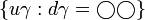$\{u\gamma:d\gamma=\bigcirc\bigcirc\}$) involving knotted trivalent graphs, edge deletions and edge unzips (and also a certain binary "connected sum" operation which we ignore here). But the algebraically defined knot invariants we now know are largely incompatible with those operations and so we can hardly expect them to yield useful information about our properties of interest.

### What We Seek - An "Algebraic Knot Theory"

To remedy this we seek more than merely knot invariants - we seek an Algebraic Knot Theory, which sees not only knots but also knotted trivalent graphs, and more importantly, which sees the edge deletion and edge unzip operations. More precisely, an Algebraic Knot Theory should consist of the following structure:

• To every trivalent graph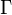$\Gamma$ some algebraically-defined space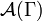${\mathcal A}(\Gamma)$ and a knotted graph invariant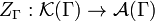$Z_\Gamma:{\mathcal K}(\Gamma)\to{\mathcal A}(\Gamma)$ (so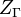$Z_\Gamma$ is an invariants of knottings of$\Gamma$ with values in${\mathcal A}(\Gamma)$).
• For every pair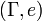$(\Gamma, e)$, where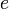$e$ is an edge of$\Gamma$, there is a pair of operations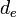$d_e$ and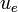$u_e$ defined on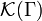${\mathcal K}(\Gamma)$ which delete (for$d_e$) or unzip (for$u_e$) the edge$e$ in any knotting of$\Gamma$. With the obvious definition of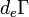$d_e\Gamma$ and of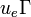$u_e\Gamma$,$d_e$ and$u_e$ take values in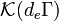${\mathcal K}(d_e\Gamma)$ and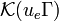${\mathcal K}(u_e\Gamma)$, respectively. In an algebraic knot theory there should be corresponding operations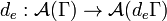$d_e:{\mathcal A}(\Gamma)\to{\mathcal A}(d_e\Gamma)$ and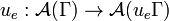$u_e:{\mathcal A}(\Gamma)\to{\mathcal A}(u_e\Gamma)$ that commute with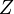$Z$ in the obvious sense.
• There should also be binary "connected sum" operations defined on pairs of spaces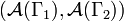$({\mathcal A}(\Gamma_1), {\mathcal A}(\Gamma_2))$, and they too should be compatible with$Z$. But in the interest of brevity, we omit the details here.

An algebraic knot theory will allow us to convert certain knot theoretical problems from topology to algebra in much of the same way that a homology functor turns certain questions in the topology of spaces to questions in algebra. For example, given an algebraic knot theory, we have that

Failed to parse (unknown function\dumbbell): Z({\mathcal R})\subset{\mathcal A}_{\mathcal R}:=\{u\zeta:\zeta\in{\mathcal A}(\dumbbell)\mbox{ and }d\zeta=Z(\bigcirc\bigcirc)\} .

Note that the right hand side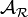${\mathcal A}_{\mathcal R}$ of this equality is belongs entirely to the world of algebra. So if the spaces and operations making up our algebraic knot theory are sufficiently well understood,${\mathcal A}_{\mathcal R}$ can be computed explicitly. But then we get a criterion for a knot to be ribbon - if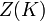$Z(K)$ isn't in${\mathcal A}_{\mathcal R}$, then$K$ isn't a ribbon knot.

Similar logic leads to similar algebraic criteria for detecting other definable properties of interest, including many (but not all) of the ones listed above. So it seems that an algebraic knot theory would be a very nice tool to have.

### The Kontsevich Integral of Knotted Trivalent Graphs

Well, are there any algebraic knot theories? The ones I know belong to either one of two classes:

• Too simple to be of use (there's an "Abelian" one, for example, built out of linking numbers).
• So hard we can't yet use them.

The former class makes for some nice examples, but otherwise there's not much to say about it. The prime member of the latter class is the Kontsevich integral, extended to knotted trivalent graphs. Such an extension exists but it is not unique; in fact, one may show that algebraic knot theories that extend the Kontsevich integral for knots and links are in a bijective correspondence with a choice of a well-behaved Drinfel'd associator. But associators are notoriously difficult to compute and to work with. It can be proven that they exist, but there is no reasonable formula for one yet. And so at least for now, the determination of sets like${\mathcal A}_{\mathcal R}$ for an algebraic knot theory extending the Kontsevich integral seems like a hopeless task. Likewise for the computation of$Z(K)$ for all but the simplest knots.

For a trivalent graph$\Gamma$, let${\mathcal A}(\Gamma)$ denote the target space of the Kontsevich integral for knotted$\Gamma$'s. (For those who know the words,${\mathcal A}(\Gamma)$ is the space of Jacobi diagrams with skeleton$\Gamma$ modulo AS, STU, IHX and vertex invariance relations). As said, at present the spaces${\mathcal A}(\Gamma)$ are too hard to understand. But they posses many interesting quotients that may well yield some useful information.

### The Envelopes of Classical Invariants

There is one particularly nice way of finding "ideals" in the "algebra"${\mathcal A}(\Gamma)$ which allows us to start from some "classical" knot invariant and its "envelope" - its minimal extension to be an algebraic knot theory.

Let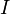$I$ be a finite type invariant or a power series of finite type invariants (such as the "classical" Jones or Alexander polynomials). Let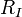$R_I$ be "internal kernel" of$I$: the collection of all "internal" relations satisfied by the weight system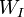$W_I$ of$I$ - these would be those linear combinations of internal parts of Jacobi diagrams which are annihilated by$W_I$ whenever they appear inside a complete Jacobi diagram in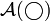${\mathcal A}(\bigcirc)$.

Trivial examples would be the AS and IHX relations as they are annihilated by any weight system (and so in a sense, there is no reason to mention them). A non-example would be the STU relation, for it explicitly involves the skeleton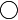$\bigcirc$ and so it is not internal. A non-trivial example is the relation Failed to parse (unknown function\HGraph): \HGraph=2\hbar(\hsmoothing-\crossing)

found inside the internal kernel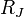$R_J$ of the Jones polynomial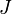$J$ (this relation is also known as the vector identity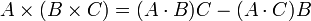$A\times(B\times C)=(A\cdot B)C-(A\cdot C)B$ and is related to the su(2)/so(3) algebra associated with the Jones polynomial).


Anyway, since the relations in$R_I$ are internal, they can be applied no matter what the skeleton is. So we can form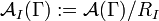${\mathcal A}_I(\Gamma):={\mathcal A}(\Gamma)/R_I$ and project the (extended) Kontsevich integral to these spaces. The result inherits the structure of an algebraic knot theory from the Kontsevich integral, and has the right to be called "the envelope of$I$", as it contains$I$ and at least in some finite-type sense, only whatever must be added to$I$ to make it an algebraic knot theory.

So far I have studied only two such envelopes in some detail.

• The envelope of the Jones polynomial seems to contain all coloured Jones polynomials of knots, links and graphs and nothing else. But unfortunately, it still involves some formulas that I cannot manage.
• The envelope of the Alexander polynomial is seductively just, really just, beyond my reach for now. I expect it to be the first useful example of an algebraic knot theory once it is fully understood.

But I must emphasize that other envelopes are around (one for each classical invariant) and that many other "internal" quotients of the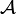${\mathcal A}$ spaces exist, that do not necessarily come as an envelope. So optimistically I expect the utility of the notion "algebraic knot theory" to reach way beyond the Alexander polynomial.

### The Challenges

• It is known that the Alexander polynomial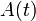$A(t)$ of a ribbon knot is always of the form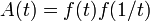$A(t)=f(t)f(1/t)$, where$f$ is some other polynomial. One of the first tests of our proposal would be whether we are able to reproduce this result. This should entail the following steps:
• Figure out the internal kernel of the Alexander polynomial. Some hints are at my paperlet The Envelope of The Alexander Polynomial.
• Figure out the envelope of the Alexander polynomial, as an algebraic "target space". What dimension is it, for various skeletons$\Gamma$? Can we describe the algebraic operations$u$ and$d$ here?
• Figure out which finite type invariants lie in the envelope of the Alexander polynomial. These will include the Alexander polynomial for knots (of course) and linking numbers (exercise), the multi-variable Alexander polynomial (almost surely) and maybe more but not much more.
• Find how to compute the projection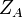$Z_A$ of$Z$ to the envelope of the Alexander polynomial. This will most likely entail finding an explicit "Alexander Associator".
• For a knotted trivalent graph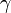$\gamma$, find in what sense exactly is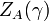$Z_A(\gamma)$ a "polynomial".
• Prove that for "polynomials"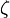$\zeta$ in Failed to parse (unknown function\dumbbell): {\mathcal A}_A(\dumbbell)
for which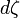$d\zeta$ is trivial,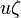$u\zeta$ if of a form appropriate for a product like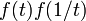$f(t)f(1/t)$. Everything in the previous sentence should be interpreted in the envelope of the Alexander polynomial; in particular,$\zeta$ will be a polynomial in the sense which will only be made explicit after answering the previous question.


(Unfortunately I run out of time to write the more interesting challenges... this will happen later).